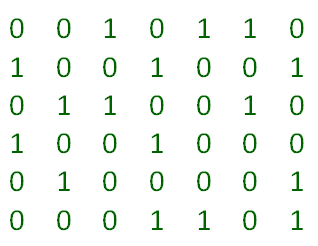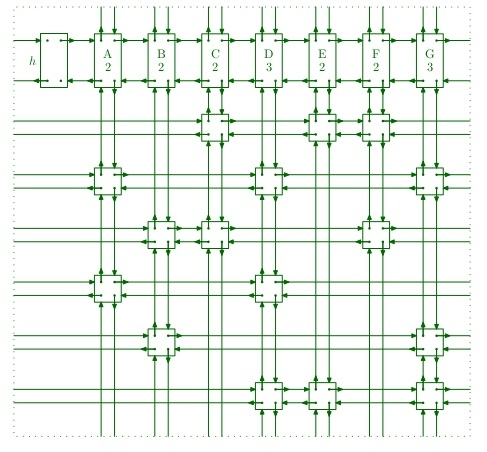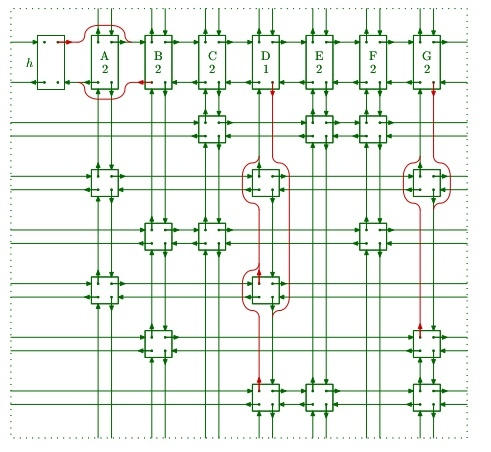# Exact Cover Problem and Algorithm X | Set 2 (Implementation with DLX)

• Difficulty Level : Hard
• Last Updated : 24 Apr, 2020

In the article Exact Cover Problem and Algorithm X | Set 1 we discussed the Exact Cover problem and Algorithm X to solve the Exact cover problem. In this article, we’ll discuss the implementation details of Algorithm X using Dancing Links Technique (DLX) proposed by Dr Donald E. Knuth in his paper “Dancing Links

Dancing link technique relies on the idea of doubly circular linked list. As discussed in previous article, we transform exact cover problem in form of matrix of 0 and 1. Here each “1” in the matrix is represented by a node of linked list and the whole matrix is transformed into a mesh of 4 way connected nodes. Each node contains following fields –

• Pointer to node left to it
• Pointer to node right to it
• Pointer to node above it
• Pointer to node below it
• Pointer to list header node to which it belongs

Each row of the matrix is thus a circular linked list linked to each other with left and right pointers and each column of the matrix will also be circular linked list linked to each above with up and down pointer. Each column list also includes a special node called “list header node”. This header node is just like simple node but have few extra fields –

• Column id
• Count of nodes in current column

We can have two different kinds of nodes but in our implementation, we will create only one kind of node with all fields for convenience with an additional “Row id” field which will tell which row this node belongs to.
So for matrix –The 4-way linked matrix will look like this –So the pseudo code for search algorithm (Algorithm X) will be –

```f( h.right == h ) {
printSolutions();
return;
}
else {
ColumnNode column = getMinColumn();
cover(column);

for( Node row = column.down ; rowNode != column ;
rowNode = rowNode.down ) {

for( Node rightNode = row.right ; rightNode != row ;
rightNode = rightNode.right )
cover( rightNode );

Search( k+1);
solutions.remove( rowNode );
column = rowNode.column;

for( Node leftNode = rowNode.left ; leftNode != row ;
leftNode = leftNode.left )
uncover( leftNode );
}
uncover( column );
}
```

Covering Node

As discussed in the algorithm, we have to remove columns and all the rows to which nodes of that column belongs to. This process is here referred as covering of node.
To remove a column we can simply unlink header of that column from neighboring headers. This way this column cannot be accessed. This process is similar to removal of node from doubly linked list, suppose we want to remove node x then –

```x.left.right = x.right
x.right.left = x.left
```

Similarly to remove a row we have to unlink all nodes of row from nodes of row above and below it.

```x.up.down = x.down
x.down.up = x.up
```

Thus the pseudo code for cover(node) becomes –

```Node column = dataNode.column;

column.right.left = column.left;
column.left.right = column.right;

for( Node row = column.down ; row != column ; row = row.down )
for( Node rightNode = row.right ; rightNode != row ;
rightNode = rightNode.right ) {
rightNode.up.down = rightNode.down;
rightNode.down.up = rightNode.up;
}
}
```

So for example, after covering column A, matrix will look like this –covering

Here we first remove the column from other columns then we move down to each column node and remove row by traversing right, so row 2 and 4 is removed.

Uncovering Node

Suppose algorithm reached a dead end and no solution is possible in that case algorithm have to backtrack. Because we have removed columns and rows when we backtrack we have link again those removed rows and columns. This is what we are calling uncovering. Notice that the removed nodes still have pointers to their neighbors, so we can link them back again using these pointers.
To uncover column we will perform covering operation but in reverse order –

```x.left.right = x
x.right.left = x
```

Similarly to uncover any row node x –

```x.up.down = x
x.down.up = x
```

Thus the pseudo code for uncover(node) will become –

```Node column = dataNode.column;

for( Node row = column.up ; row != column ; row = row.up )
for( Node leftNode = row.left ; leftNode != row ;
leftNode = leftNode.right ) {
leftNode.up.down = leftNode;
leftNode.down.up = leftNode;
}
column.right.left = column;
column.left.right = column;
}
```

Following is the implementation of dancing link technique –

 `// C++ program for solving exact cover problem ` `// using DLX (Dancing Links) technique ` ` `  `#include ` ` `  `#define MAX_ROW 100 ` `#define MAX_COL 100 ` ` `  `using` `namespace` `std; ` ` `  `struct` `Node ` `{ ` `public``: ` `    ``struct` `Node *left; ` `    ``struct` `Node *right; ` `    ``struct` `Node *up; ` `    ``struct` `Node *down; ` `    ``struct` `Node *column; ` `    ``int` `rowID; ` `    ``int` `colID; ` `    ``int` `nodeCount; ` `}; ` ` `  `// Header node, contains pointer to the ` `// list header node of first column ` `struct` `Node *header = ``new` `Node(); ` ` `  `// Matrix to contain nodes of linked mesh ` `struct` `Node Matrix[MAX_ROW][MAX_COL]; ` ` `  `// Problem Matrix ` `bool` `ProbMat[MAX_ROW][MAX_COL]; ` ` `  `// vector containing solutions ` `vector <``struct` `Node*> solutions; ` ` `  `// Number of rows and columns in problem matrix  ` `int` `nRow = 0,nCol = 0; ` ` `  ` `  `// Functions to get next index in any direction ` `// for given index (circular in nature)  ` `int` `getRight(``int` `i){``return` `(i+1) % nCol; } ` `int` `getLeft(``int` `i){``return` `(i-1 < 0) ? nCol-1 : i-1 ; } ` `int` `getUp(``int` `i){``return` `(i-1 < 0) ? nRow : i-1 ; }   ` `int` `getDown(``int` `i){``return` `(i+1) % (nRow+1); } ` ` `  `// Create 4 way linked matrix of nodes ` `// called Toroidal due to resemblance to ` `// toroid ` `Node *createToridolMatrix() ` `{ ` `    ``// One extra row for list header nodes ` `    ``// for each column ` `    ``for``(``int` `i = 0; i <= nRow; i++) ` `    ``{ ` `        ``for``(``int` `j = 0; j < nCol; j++) ` `        ``{ ` `            ``// If it's 1 in the problem matrix then  ` `            ``// only create a node  ` `            ``if``(ProbMat[i][j]) ` `            ``{ ` `                ``int` `a, b; ` ` `  `                ``// If it's 1, other than 1 in 0th row ` `                ``// then count it as node of column  ` `                ``// and increment node count in column header ` `                ``if``(i) Matrix[j].nodeCount += 1; ` ` `  `                ``// Add pointer to column header for this  ` `                ``// column node ` `                ``Matrix[i][j].column = &Matrix[j]; ` ` `  `                ``// set row and column id of this node ` `                ``Matrix[i][j].rowID = i; ` `                ``Matrix[i][j].colID = j; ` ` `  `                ``// Link the node with neighbors ` ` `  `                ``// Left pointer ` `                ``a = i; b = j; ` `                ``do``{ b = getLeft(b); } ``while``(!ProbMat[a][b] && b != j); ` `                ``Matrix[i][j].left = &Matrix[i][b]; ` ` `  `                ``// Right pointer ` `                ``a = i; b = j; ` `                ``do` `{ b = getRight(b); } ``while``(!ProbMat[a][b] && b != j); ` `                ``Matrix[i][j].right = &Matrix[i][b]; ` ` `  `                ``// Up pointer ` `                ``a = i; b = j; ` `                ``do` `{ a = getUp(a); } ``while``(!ProbMat[a][b] && a != i); ` `                ``Matrix[i][j].up = &Matrix[a][j]; ` ` `  `                ``// Down pointer ` `                ``a = i; b = j; ` `                ``do` `{ a = getDown(a); } ``while``(!ProbMat[a][b] && a != i); ` `                ``Matrix[i][j].down = &Matrix[a][j]; ` `            ``} ` `        ``} ` `    ``} ` ` `  `    ``// link header right pointer to column  ` `    ``// header of first column  ` `    ``header->right = &Matrix; ` ` `  `    ``// link header left pointer to column  ` `    ``// header of last column  ` `    ``header->left = &Matrix[nCol-1]; ` ` `  `    ``Matrix.left = header; ` `    ``Matrix[nCol-1].right = header; ` `    ``return` `header; ` `} ` ` `  `// Cover the given node completely ` `void` `cover(``struct` `Node *targetNode) ` `{ ` `    ``struct` `Node *row, *rightNode; ` ` `  `    ``// get the pointer to the header of column ` `    ``// to which this node belong  ` `    ``struct` `Node *colNode = targetNode->column; ` ` `  `    ``// unlink column header from it's neighbors ` `    ``colNode->left->right = colNode->right; ` `    ``colNode->right->left = colNode->left; ` ` `  `    ``// Move down the column and remove each row ` `    ``// by traversing right ` `    ``for``(row = colNode->down; row != colNode; row = row->down) ` `    ``{ ` `        ``for``(rightNode = row->right; rightNode != row; ` `            ``rightNode = rightNode->right) ` `        ``{ ` `            ``rightNode->up->down = rightNode->down; ` `            ``rightNode->down->up = rightNode->up; ` ` `  `            ``// after unlinking row node, decrement the ` `            ``// node count in column header ` `            ``Matrix[rightNode->colID].nodeCount -= 1; ` `        ``} ` `    ``} ` `} ` ` `  `// Uncover the given node completely ` `void` `uncover(``struct` `Node *targetNode) ` `{ ` `    ``struct` `Node *rowNode, *leftNode; ` ` `  `    ``// get the pointer to the header of column ` `    ``// to which this node belong  ` `    ``struct` `Node *colNode = targetNode->column; ` ` `  `    ``// Move down the column and link back ` `    ``// each row by traversing left ` `    ``for``(rowNode = colNode->up; rowNode != colNode; rowNode = rowNode->up) ` `    ``{ ` `        ``for``(leftNode = rowNode->left; leftNode != rowNode; ` `            ``leftNode = leftNode->left) ` `        ``{ ` `            ``leftNode->up->down = leftNode; ` `            ``leftNode->down->up = leftNode; ` ` `  `            ``// after linking row node, increment the ` `            ``// node count in column header ` `            ``Matrix[leftNode->colID].nodeCount += 1; ` `        ``} ` `    ``} ` ` `  `    ``// link the column header from it's neighbors ` `    ``colNode->left->right = colNode; ` `    ``colNode->right->left = colNode; ` `} ` ` `  `// Traverse column headers right and  ` `// return the column having minimum  ` `// node count ` `Node *getMinColumn() ` `{ ` `    ``struct` `Node *h = header; ` `    ``struct` `Node *min_col = h->right; ` `    ``h = h->right->right; ` `    ``do` `    ``{ ` `        ``if``(h->nodeCount < min_col->nodeCount) ` `        ``{ ` `            ``min_col = h; ` `        ``} ` `        ``h = h->right; ` `    ``}``while``(h != header); ` ` `  `    ``return` `min_col; ` `} ` ` `  ` `  `void` `printSolutions() ` `{ ` `    ``cout<<``"Printing Solutions: "``; ` `    ``vector<``struct` `Node*>::iterator i; ` ` `  `    ``for``(i = solutions.begin(); i!=solutions.end(); i++) ` `        ``cout<<(*i)->rowID<<``" "``; ` `    ``cout<<``"\n"``; ` `} ` ` `  `// Search for exact covers ` `void` `search(``int` `k) ` `{ ` `    ``struct` `Node *rowNode; ` `    ``struct` `Node *rightNode; ` `    ``struct` `Node *leftNode; ` `    ``struct` `Node *column; ` ` `  `    ``// if no column left, then we must ` `    ``// have found the solution ` `    ``if``(header->right == header) ` `    ``{ ` `        ``printSolutions(); ` `        ``return``; ` `    ``} ` ` `  `    ``// choose column deterministically ` `    ``column = getMinColumn(); ` ` `  `    ``// cover chosen column ` `    ``cover(column); ` ` `  `    ``for``(rowNode = column->down; rowNode != column;  ` `        ``rowNode = rowNode->down ) ` `    ``{ ` `        ``solutions.push_back(rowNode); ` ` `  `        ``for``(rightNode = rowNode->right; rightNode != rowNode; ` `            ``rightNode = rightNode->right) ` `            ``cover(rightNode); ` ` `  `        ``// move to level k+1 (recursively) ` `        ``search(k+1); ` ` `  `        ``// if solution in not possible, backtrack (uncover) ` `        ``// and remove the selected row (set) from solution ` `        ``solutions.pop_back(); ` ` `  `        ``column = rowNode->column; ` `        ``for``(leftNode = rowNode->left; leftNode != rowNode; ` `            ``leftNode = leftNode->left) ` `            ``uncover(leftNode); ` `    ``} ` ` `  `    ``uncover(column); ` `} ` ` `  `// Driver code ` `int` `main() ` `{     ` `    ``/* ` `     ``Example problem ` ` `  `     ``X = {1,2,3,4,5,6,7} ` `     ``set-1 = {1,4,7} ` `     ``set-2 = {1,4} ` `     ``set-3 = {4,5,7} ` `     ``set-4 = {3,5,6} ` `     ``set-5 = {2,3,6,7} ` `     ``set-6 = {2,7} ` `     ``set-7 = {1,4} ` ` `  `     ``Solutions : {6 ,4, 2} and {6, 4, 7} ` `    ``*/` ` `  `    ``nRow = 7; ` `    ``nCol = 7; ` `     `  `    ``// initialize the problem matrix  ` `    ``// ( matrix of 0 and 1) with 0 ` `    ``for``(``int` `i=0; i<=nRow; i++) ` `    ``{ ` `        ``for``(``int` `j=0; j

Output:

```Printing Solutions: 6 4 2
Printing Solutions: 6 4 7
```

References

This article is contributed by Atul Kumar. If you like GeeksforGeeks and would like to contribute, you can also write an article using contribute.geeksforgeeks.org or mail your article to contribute@geeksforgeeks.org. See your article appearing on the GeeksforGeeks main page and help other Geeks.